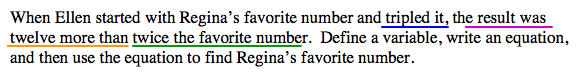### Home > AC > Chapter 4 > Lesson 4.2.4 > Problem4-105

4-105.

When Ellen started with Regina's favorite number and tripled it, the result was twelve more than twice the favorite number. Define a variable, write an equation, and then use the equation to find Regina's favorite number.

Define a variable.

$r$ = Regina's favorite number

Use the first sentence to write an equation.

Look at the important parts of the problem above.$\color{blue}{3r} \color{purple}{=} \color{orange}{12} + \color{green}{2r}$

Solve for $r$.